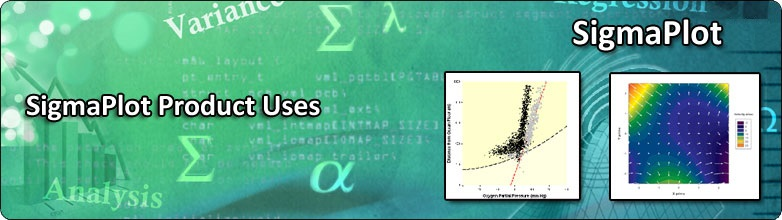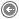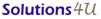, SSIGMAPLOT PRODUCT USES

 Macro Concepts Transforms Graphs Analyses Data Smoothing Controlled Release Analysis SigmaPlot & Excel SigmaPlot & Matlab Data Formats Curve Fitting

The following whitepapers highlight these application areas:

A. Macro Concepts

a. Creating a Macro

i. Recording a Macro - A tutorial showing how to record a macro that fits a decaying exponential curve to two data sets. The macro is then modified by 1) adding a for-loop, 2) adding a dialog to allow user control and 3) placing the macro name on the main menu.

ii. Creating a New Menu Item and Adding a Macro Name To It

iii. Run a Macro from the Toolbox Menu - Descriptions of the macros in the SigmaPlot Toolbox menu item.

v. Adding a Dialog to a Macro - Describes how to create a dialog interface to a macro.

vi. Important Issues With Recorded Macros

1. Making the Graph Page the Currently Displayed Window

2. Using Statements from Recorded Macros
b. Batch Processing using SigmaPlot Automation - Select a group of Excel files to process in a batch. This macro fits a user-selected SigmaPlot curve fit equation to the data, creates a graph and saves the results for all Excel files in a SigmaPlot notebook.

c. SigmaPlot Macro Sample Code - Useful macro sample code is provided for the user to copy.

Scale Graph Macro

B. Transforms

a. Rowwise Statistics Transform - This transform computes rowwise statistics. It complements the columnwise statistics available from the main menu.

b. Transform Functions root and implicit - These two new functions have been added to solve equations.

C. Graphs

a. 2D Histogram - Histogram and cumulative histograms are created from a single column of data. Four graph types and other options are provided.

b. 3D Histogram - A three-dimensional bar-graph histogram is created from bivariate data.

c. Asymmetric Error Bars - This macro creates one of three types of graphs with asymmetric error bars from relative error bar data.

d. Preparing Your Graphs for Journal or Web Publication - A discussion of the graph file formats used and/or required for journal and web publication.

e. Quality Control Charts - Xbar and Range charts are created using the SigmaPlot Reference Line feature.

f. Ribbon Graph - This transform uses XZ profiles from XY Many Z data to generate the individual ribbons of a ribbon graph. This transform is located in your SigmaPlot Transforms folder.

g. Shade Between Two Curves - This macro creates a shade between two curves. It complements SigmaPlot’s built-in area plot feature that shades area under the curve to the X-axis. The macro assumes the data for both curves is strictly increasing in x.

h. Formatted SigmaPlot graphs for Submission to the FDA - The procedure is described for pasting SigmaPlot graphs into Microsoft Word that fit within specified margins and have a fixed font size.

D. Analyses

a. Analysis of Ligand Binding Data - Competition, saturation and dose-response studies may be analyzed with SigmaPlot Version 7.0 and this macro. Multiple replicate data sets are fit using an equation selected from a list of ten – and you may add your own. Graphical results, EC50 values and a statistical report are produced.

b. Analyzing Dissolution Test Data with SigmaPlot’s Excel Spreadsheet - An Excel worksheet analyzes up to 12 vessel by 6 sample time dissolution test data. Publication quality graphs of the results are simultaneously created.

c. Shelf Life Time Analysis

i. Computing Shelf Life Time with SigmaPlot - An exact computation of shelf life time is computed and graph created. Four designs are available – lower specification only, upper specification only, lower and upper specification and degradant analysis.

ii. Validation of the Shelf Life Macro - Confirms accuracy of the Shelf Life Macro

iii. Simulation

iv. Transform

d. Data Smoothing - Three real-world examples with increasing variability show the usefulness of SigmaPlot’s data smoothing algorithms to visualize the information in the data. [top]

e. Controlled Release Analysis

i. Fitting Controlled Release and Dissolution Data - Five controlled release models for analysis of drug dissolution data are implemented as a SigmaPlot fit library. One or all models may be easily fitted to your data.

ii. Explicit Function Approximation

iii. Create These Functions

iv. Modify Equations in the SigmaPlot Fit Library

f. Global Analysis of Concentration Response Curves - Global curve fitting without data concatenation is demonstrated.

g. Global Curve Fit of Enzyme Kinetics Data - A demonstration of simultaneous fitting of multiple functions to multiple data sets with shared parameters.

h. Global Curve Fitting for Ka and Kd from Sedimentation - A global analysis of ultracentrifuge radial macromolecule concentration gradients.

i. Global Curve Fitting - Dose Response Parallelism

j. Curve Fitting / Regression

k. Piecewise Nonlinear Regression - A four-segment piecewise linear equation is fit to rowwise replicate data.

l. Weight Functions in Nonlinear Regression

E. SigmaPlot & Excel

a. Create a SigmaPlot Graph in Excel - An Excel macro that creates a SigmaPlot graph in Excel.

b. Using macros to place SigmaPlot charts in Microsoft - The macro statements required to insert a SigmaPlot graph into a PowerPoint slide are shown and explained. The similar procedure for placing a graph in Word is then shown.

F. SigmaPlot & MatLab

G. Data Formats

a. Replicate Data Format

b. X,Y Many Z to X, Y, Z Format - This transform converts from one format to another. For example, the 3D smoothing algorithms requires data in XYZ format.MY Office : 72-3C, JALAN PUTERI 2/4, BANDAR PUTERI, 47100 PUCHONG, SELANGOR, Malaysia. Tel:+603-8063 9300 fax:+603-8063 9400 SG Office : 259, Onan Road, Singapore 424651. Tel: +65-6468 3325 Fax: +65-6764 5646